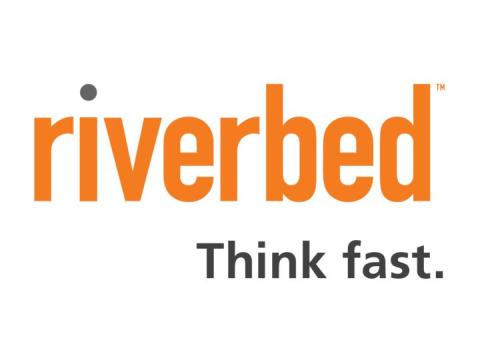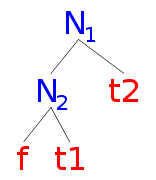## Me## What's the Point?

• Share an interesting data structure
• Show some Scala code

Remind you to ignore math

## What is a Zipper?

• Reification of a walk
• Derivative of a data structure
• A data structure with a hole
• Just something useful

## Something Useful

It allows us to navigate a data structure and modify components as we go along

"Solving" the in-place edit "problem"

## Something Useful

• O(1) - In Place Update

vs

• O(n)/O(log n) - Update

## Something Useful

Take a List[T],

we can make a Zipper of form,

(before: List[T], focus: T, after: List[T])

## Example 1

val ls = List('a','b','c','d','e')

if 'd' is the focus

val zipper = (['c','b','a'],'d',['e'])

## Zipped List

```case class ZippedList[T](before: List[T], focus: T,
after: List[T]) {
def goLeft(): ZippedList[T] = before match {
case Nil => throw new Exception("Can't move left")
case x::xs => ZippedList(xs,x,focus::after)
}
def goRight(): ZippedList[T] = after match {
case Nil => throw new Exception("Can't move right")
case x::xs => ZippedList(focus::before,x,xs)
}
def update(f: T => T): ZippedList[T] =
ZippedList(before,f(focus),after)
def zipUp(): List[T] = (focus :: before).reverse ++ after
}
```

## Form

PATH, FOCUS, REST

## Trees

Take a binary tree with values,

```abstract class BTree[A]
case class Leaf[A](value: A) extends BTree[A]
case class Node[A](value: A, left: BTree[A],
right: BTree[A]) extends BTree[A]
```

## Zipper

Simplify to Path, Focus

```case class ZippedTree[T](path: List[Decision[BTree[T]]],
focus: BTree[T])
```

## Go Left

```def goLeft() : ZippedTree[T] = focus match {
case Leaf(_) => throw new Exception("Can't move left")
case Node(_,l,_) => ZippedTree(Left(focus)::path,l)
}
```

## Defs

```abstract class Decision[T]
case class Left[T](value: T) extends Decision[T]
case class Right[T](value: T) extends Decision[T]
```

## Go Right

```def goRight() : ZippedTree[T] = focus match {
case Leaf(_) => throw new Exception("Can't move right")
case Node(_,_,r) => ZippedTree(Right(focus)::path,r)
}
```

## Go Up

```def goUp() : ZippedTree[T] = path match {
case Nil => throw new Exception("Can't go up")
case Left(Node(v,_,r)) :: rest =>
ZippedTree(rest,Node(v,focus,r))
case Right(Node(v,l,_)) :: rest =>
ZippedTree(rest,Node(v,l,focus))
}
```

## Update

```def update(f: BTree[T] => BTree[T]): ZippedTree[T] =
ZippedTree(path,f(focus))
```

## ZipUP

```def zipUp(): BTree[T] = {
def rollup(z: ZippedTree[T]): BTree[T] = z match {
case ZippedTree(Nil,f) => f
case x => rollup(x.goUp())
}
rollup(this)
}
```

## Example

```val t: BTree[Int] = Node(1,
Node(7,
Leaf(3),
Leaf(4)),
Node(7,
Node(7,
Leaf(9),
Leaf(2)),
Leaf(4)))
```

## Example...## What else can we Zip?

• N-ary Trees
• Streams
• ...any simple sum, product or recursive type

## Maths interlude...

What are we doing?

Really all about creating paths to the current focus

## Maths interlude...

Bit more formality

Path is a list of decisions, so let's annotate our decision and store the siblings of a node in the tree.

## Maths interlude...path to f is;

Left(N2) :: Left(N1)

## Maths interlude...

Left(N2) :: Left(N1) + f => ZIPPER

Since we can recover the tree from the Zipper,

Left(N2) :: Left(N1) is the tree minus f,

the tree with a hole

## One hole contexts

Left(N2) :: Left(N1)

That's a one hole context of BTree,

where BTree is a product type of Leaf | BTree*BTree

The notation * indicates a product type.
Think any tree with any other tree

You can do the same for ternary trees
Leaf | TTree*TTree*TTree

## One hole contexts...

Each decision we make in a BTree has the type

Decision[Btree] which can be expressed
Left(BTree) | Right(BTree)

Which happens to be a sum type, BTree+BTree

Think all lefts and all rights

## One hole contexts...

So a generous rewriting may give us,

BTree = 1 + BTree^2

TTree = 1 + TTree^3

∂BTree = 2BTree

∂TTree = 3TTree …

## One hole contexts...

The derivative of a regular type is its type of one-hole contexts

## So...?

In theory it is possible to synthesise a Zipper from any regular type, with arbitrary navigation and update.

## So...?

In practise we need more type-level programing...